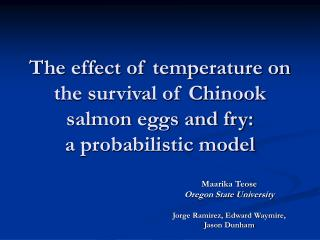DownloadDownload PresentationThe effect of temperature on the survival of Chinook salmon eggs and fry: a probabilistic model

# The effect of temperature on the survival of Chinook salmon eggs and fry: a probabilistic model

Download Presentation## The effect of temperature on the survival of Chinook salmon eggs and fry: a probabilistic model

- - - - - - - - - - - - - - - - - - - - - - - - - - - E N D - - - - - - - - - - - - - - - - - - - - - - - - - - -
##### Presentation Transcript

1. The effect of temperature on the survival of Chinook salmon eggs and fry: a probabilistic model Maarika Teose Oregon State University Jorge Ramirez, Edward Waymire, Jason Dunham

2. Background – Cougar Dam • Location • ESA-Listed Chinook Salmon • Temperature Control Structure http://www.bpa.gov/corporate/BPANews/Library/images/Dams/Cougar.jpg

3. Background - Salmon • Early Life History • Spawning, Egg, Alevin, Fry • Effect of Temperature • Studied exhaustively • Some equations exist • “Egg-Fry Conflict” (Quinn 2005) http://wdfw.wa.gov/wildwatch/salmoncam/hatchery.html

4. Background - Intention • Qualitative model • Incubation temperature (T) vs. rearing temperature (T2) • Survival and fitness of salmon

5. Construction - Objective Measure of fitness: Biomass Biomass = avg. weight × pop. size pop. size = (# eggs laid) × P(E) where P(E) = probability that an egg survives to hatching

6. Construction - Objective N = # eggs in reach P(E) = Probability that an egg hatches E(W|E) = Expected weight (i.e. average weight) given that the egg hatched Biomass = E(W|E) × N × P(E) It remains to find E(W|E)

7. Th Construction - Objective Weight tm Time

8. Construction • Fish weight at time tm= W(t,T2) (Elliott & Hurley 1997) • Amount of time the fish grows (t) • Rearing temperature (T2) Need an expression for the amount of time a fish has to grow.

9. Construction • Recall Th has a density function: fTh(t,T) • Equation for median hatching time (Crisp 2000): D2(T) • D2(T) determines location of fTh(t,T)

10. Construction Tg = amt of time a fish has to grow before tm Tg = tm – Th Median of distribution of Tg given by tm – D2(T) • Probability density function vTg(t,T) • Cumulative distribution function VTg(t,T)

11. Construction Recall: • Cumulative Distribution Function “G(x)” G(x) = P(X ≤ x) • In our case VTg(t,T) = P(Tg ≤ t) Probability that for some incubation temperature T, the time the fish has to grow once it hatches is less than t.

12. Construction • Notice: P(W ≤ w)=P(Tg ≤ z) • Solve W(t,T2) for time • New expression: z(w,T2) Gives time needed to grow to w grams when reared at temperature T2 = VTg(z(w,T2),T)

13. Construction Formula for Expected Value:

14. Results • Let Th, Tg have symmetrical triangular distributions • Assume no fry mortality

15. Results • P(E)=H(T) Fit curve to data (Current function is a very poor fit) • N = #eggs Fecundity: ~2000-17,000

16. Results • Biomass B(T,T2)= E(W|E) × N × P(E)

17. Results – Cougar Dam • USGS water temperature gauges • Above reservoir (14159200) • Below dam (14159500) • According to current model: • Temp regime above reservoir → 110.7 kg • Temp regime below dam → 156 kg • By current model, dam encourages growth and survival!

18. Conclusion • Improvements: • Realistic distribution for Th, Tg • Introduce fry mortality into model • Improved form of H(T) • Further research: • Is T or T2 more decisive in determining a population’s biomass? • What is the implication of one generation’s biomass on successive generations?

19. Eco-Informatics • Eco-informatics in my project • Fish biology • Probability theory • Maple 10 • Other discipline: Statistics

20. Acknowledgements Thanks to Jorge Ramirez, Jason Dunham, Edward Waymire, Desiree Tullos and the 2007 Eco-Informatics Summer Institute, everyone at the HJ Andrews Experimental Forest, and the National Science Foundation. • References Crisp, D.T. (2000). Trout and salmon: ecology, conservation and rehabilitation. Oxford, England: Blackwell Science. Elliott, J.M., & Hurley, M.A. (1997). A functional model for maximum growth of Atlantic salmon parr, salmo salar, from two populations in northwest England. Functional Ecology. 11, 592-603. Quinn, Tom (2005). The behavior and ecology of Pacific salmon and trout. Seattle, WA: University of Washington Press.